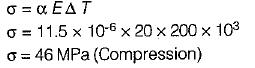Courses

# Test: Tension, Compression & Shear - 2

## 10 Questions MCQ Test Topicwise Question Bank for Mechanical Engineering | Test: Tension, Compression & Shear - 2

Description
This mock test of Test: Tension, Compression & Shear - 2 for Mechanical Engineering helps you for every Mechanical Engineering entrance exam. This contains 10 Multiple Choice Questions for Mechanical Engineering Test: Tension, Compression & Shear - 2 (mcq) to study with solutions a complete question bank. The solved questions answers in this Test: Tension, Compression & Shear - 2 quiz give you a good mix of easy questions and tough questions. Mechanical Engineering students definitely take this Test: Tension, Compression & Shear - 2 exercise for a better result in the exam. You can find other Test: Tension, Compression & Shear - 2 extra questions, long questions & short questions for Mechanical Engineering on EduRev as well by searching above.
QUESTION: 1

### A member having length L, cross-sectional area A and modulus of elasticity E is subjected to an axial load W. The strain energy stored in this member is

Solution: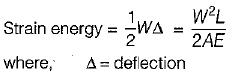QUESTION: 2

### A straight bar having a constant cross-sectional area A, length L and weight W, is hanging vertically. If E is the Young’s modulus of the material of the bar, total increase in length of the bar due to its own weight only will be

Solution: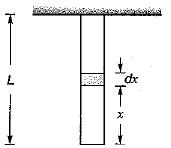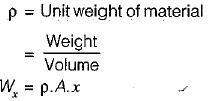Deflection of elemental length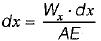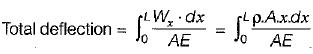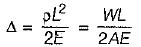QUESTION: 3

### A solid metal bar of uniform diameter D and length L is hung vertically from a ceiling. If the density of the material of the bar is p and the modulus of elasticity is E, then the total elongation of the bar due to its own weight is

Solution:

The elongation of bar due to its own weight (W) is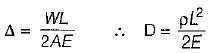where,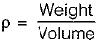QUESTION: 4

The deformation of a bar under its own weight as compared to that when subjected to a direct axial load equal to its own weight will be

Solution:

Deflection due to direct axial load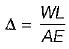Hence, Deflection due to self weight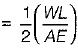= 1/2 (Deflection due to direct axial load)

QUESTION: 5

A tapering bar (diameter of end sections being, d1 and d2) and a bar of uniform cross-section, 'd' have the same length and are subjected to the same axial pull. Both the bars will have the same extension if ‘d’ is equal to

Solution: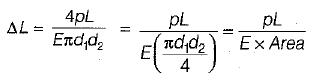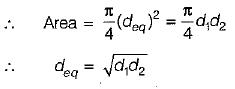QUESTION: 6

The elongation of a conical bar under its own weight is equal to

Solution: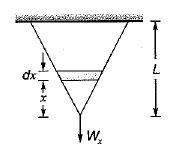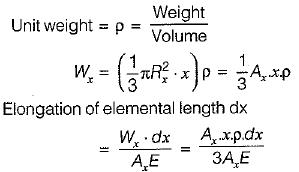Total elongation of bar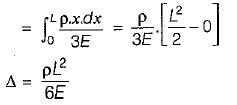Elongation of prismatic bar due to self weight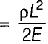Elongation of conical bar due to self weight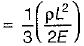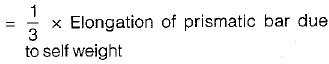QUESTION: 7

A 100 mm x 5 mm steel bar free to expand is heated from 15°C to 40°C. What shall be induced in the bar after heating?

Solution:

If a body is allowed to expand or contract freely with rise or fall of temperature then no stresses are induced in the body. If this expansion or contraction is prevented then internal resisting forces are developed. The stresses caused by these internal forces are known as thermal stresses.

QUESTION: 8

A rod of material with E = 200 x 103 MPa and α = 10-3/°C is fixed at both the ends. It is uniformly heated such that the increase in temperature is 30°C. The stress developed in the rod is

Solution:

Thermal stress (σ) = αEΔT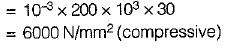The stress induced is compressive in nature as a compressive force is exerted by the supports which prevent increase in length due to increase is temperature.

QUESTION: 9

A cube having each side of length a, is constrained in all directions and is heated uniformly so that the temperature is raised to T°C. If α is the thermal coefficient of expansion of the cube material and E is the modulus of elasticity, the stress developed in the cube is

Solution:

Since the cube is constrainted in all directions therefore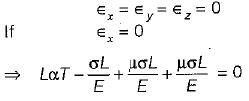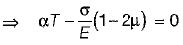⇒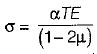QUESTION: 10

A steel rod of length 300 mm is held between two fixed supports so that the rod cannot elongate or contract in the axial direction. If the temperature of the rod is raised by 20°C, the axial stress induced in the rod due to this rise in temperature is
(Take E = 200 GPa, α = 11.5 x 10-6/°C)

Solution: